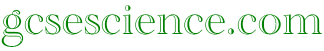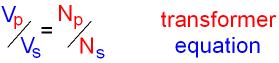# GCSE Physics Equations

## A complete list of equations for GCSE Physics Exam (All Calculations »)

This is a list of the equations which you might need to use for
your GCSE Physics Exam. Click on the link for more information.
At the bottom of the page there are links to equations
which the different examination boards have used in the past.
These links will take you to a page which you can
print if you want to so that you can revise these equations.

Electricity

P = V x I.    power = voltage x current.

V = I x R.    voltage = current x resistance.

Q = I x t.     charge = current x time.

E = V x Q.     energy = voltage x charge.

E = V x I x t.     energy = voltage x current x time.Total cost  =  number of units x cost per unit.

Energy

Efficiency (%)  =  (useful energy out ÷ total energy in) x 100.

GPE = mgh.      GPE = mass x gravity x height.

KE  =  ½mv2.     Kinetic Energy = 0·5 x mass x velocity2.

W = F x d.      work done = force x distance.

W = E.      work done = energy transferred.

P = E ÷ t.      power = energy ÷ time.

E = c x m x θ.   energy = specific heat capacity x mass x change in temperature.

Forces and Motion

s = d ÷ t.     speed = distance ÷ time.

a = (v-u) ÷ t.      acceleration = change in velocity ÷ time.

F = m x a.      Force = mass x acceleration.

w = m x g.     weight = mass x gravity.

p = m x v.     momentum = mass x velocity.

(mv - mu) = F x t.     change in momentum = Force x time.

d = mv.     density = mass ÷ volume.

p = F ÷ a.     pressure = force ÷ area.

m = F x d.     moment = force x perpendicular distance from pivot.

Waves

v = f x λ.     velocity  =  frequency wavelength.

 AQA Foundation Higher Edexcel Foundation Higher OCR Foundation Higher Wales Foundation Higher Northern Ireland Foundation Highergcsescience.com        GCSE Physics        Calculations        Index        gcsescience.com